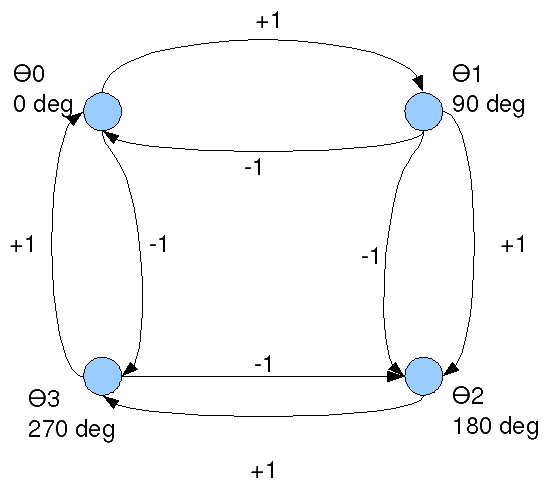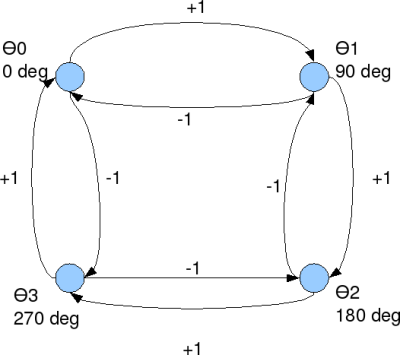# Simulating Minimum Shift Keying TransmitterMinimum shift keying (MSK) is an important concept to learn in digital communications. It is a form of continuous phase frequency shift keying . In minimum phase shift keying, two key concepts are used.

(a) The frequency separation of the sinusoidals used for representing bits 1’s and 0’s are , where is the symbol period.

(b) It is ensured that the resulting waveform is phase continuous.

## Motivation of continuous phase

In a previous post (here), we have understood that the minimum frequency separation for two sinusoidals having zero phase difference to be orthogonal is , where is the symbol period. However, it can be observed that at each symbol boundary, there is a phase discontinuity. The presence of phase discontinuities can result in large spectral side lobes outside the desired bandwidth. Hence the need for having a frequency modulated signal which is phase continuous.

## Phase growth in MSK

To ensure continuous phase, the phase of the carrier of the MSK signal is
, where

is the symbol period,
corresponds to -1 for bit 0, +1 for bit 1 respectively.

The corresponding carrier signal is
.Figure: Phase transition diagram for MSK (Ref: Fig10.22 in [COMM-PS]

## Simulation Model

Simple Octave/Matlab code for simulating and plotting binary Minimum Shift Keying is kept here.

## Next steps

We have observed that bit error probability of classical coherent binary frequency shift keying is 3dB poorer compared to bit error probability of binary phase shift keying. However, in minimum shift keying, using the knowledge of the phase transitions, we should be able to recover the 3dB loss associated with FSK and get a performance comparable to BPSK. We will hopefully discuss that in a future post.

## 17 thoughts on “Simulating Minimum Shift Keying Transmitter”

1.ABHIJEET says:

i want matlab code for reduction of ICI in ofdm using raised cosine pulse.
please can you send it my email id.
thanks

2.riki says:

Hi Krishna, can you show examples for OQPSK with sine pulse shaping using modem.oqpskmod and modem.oqpskdemod? thanks!

1.Krishna Sankar says:

@riki: Sorry, I do not have Matlab installed in my home computer.

3.Denis says:

I found my problem, it sufficed to use a coefficient of Rayleigh for 1 bit and not for the 8 samples, thank you anyway for the help

1.Krishna Sankar says:

@Denis: Ok

4.baljit says:

sir could u pls snd me matlab code for msk+convolution using awgn channel

5.Denis says:

Sorry to ask again, but have you an explanation of the difference found in relation to the theory for the simulation of MSK modulation in a Rayleigh channel, thank you

6.Denis says:

First, Thanks for your help !!

Now I try to simulate MSK (and FSK) transmission on a rayleigh channel.
With your exemple at : BER for BPSK in Rayleigh channel, I do the same thing with the MSK /FSK modulation but the results are different from theory (There is a small gap)
Can you explain it, do you have the same gap?
Thank you again for your help!

7.Denis says:

In fact, I try to program in C + +, the MSK modulation, your Matlab model is very interesting however I have some difficulties in receiving, the convolution does not work very well. Have you an idea how to demodulate the signal by taking into account the phase change?
I think that I perform the convolution in reception is not suitable, I convolves my outgoing signal channel signals with AWGN: f1 = sqrt (2 / T) * cos (2 * PI * (k +1 / 4T ) * t) and f2 = sqrt (2 / T) * cos (2 * PI * (fc-1/4T) * t).
But the results between the two convolutions are not different enough to determine correctly received bits.

What do you think?

8.Denis says:

Hi,

Also can you explain me, how you do to demodulate the signal, I have difficulties to demodulate correctly because I do not take into account the phase to the convolution of signal reception. It is difficult because we have 1 phase for each point (fsamp in reality) of the signal.

Thanks for your help and sorry for my english !

1.Krishna Sankar says:

@Denis: Sorry, I do not quite understand the concern. Did you take a look at the Matlab model in the post.

9.Hans-Werner says:

Ok, but how does the receiver looks like ?
What about carrier and phase recovery ?
How I get my signal back ?

1.Krishna Sankar says:

@Hans-Werner: I have a follow up post on the MSK receiver @